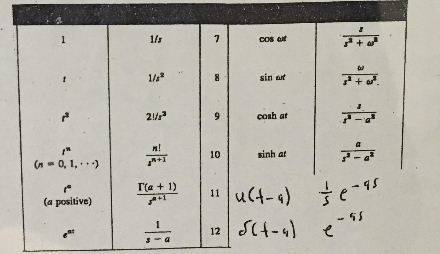Initial Value Problem using Laplace Transform help?

Homework Statement

Use laplace transforms to find following initial value problem -- there is no credit for partial fractions. (i assume my teach is against using it..)

y'' - 4y' + 3y = 0 ; y(0)=2 y'(0) = 8

Homework EquationsLf'' = ((s^2)*F) - s*f(0) - f'(0)
Lf' = sF - f(0)
Lf = F(s)

The Attempt at a Solution

My first attempt is of course realizing that the above equation is expressed in terms of 't'. So I must take the laplace transform on both sides.

L(y'') - 4 L(y') + 3L(y) = L(0)

[((s^2)*F) - s*f(0) - f'(0)] - 4 [ sF - f(0) ] + 3 [F(s)] = 0 (substituted with the above equations)

(s^2)*F - 2s - 8 - 4s*F(s) + 8 + 3*F(s) = 0 (plugged in the initial values)

Y(s)*[s^2 - 4s + 3] - 8 = 0

Y(s) = 8 / [s^2 - 4s + 3]

Knowing that s^2 + as + b = (s + a/2 ) ^ 2 + b - ((a^2)/4)
I get: (s-2)^2 - 1 for the denominator.

Y(s) = 8 / [(s-2)^2 - 1 ]

At this final step....I am not sure how I will be able to transform to Y(t) again if I am not allowed to use "Partial Fractions"? Any assistance would be truly appreciated. Thanks!

The solution for this problem is :
y = 2(e^2t)*cosht + 4(e^2t)*sinht

Last edited:

Ray Vickson
Homework Helper
Dearly Missed

Homework Statement

Use laplace transforms to find following initial value problem -- there is no credit for partial fractions. (i assume my teach is against using it..)

y'' - 4y' + 3y = 0 ; y(0)=2 y'(0) = 8

Homework Equations

View attachment 91182
Lf'' = ((s^2)*F) - s*f(0) - f'(0)
Lf' = sF - f(0)
Lf = F(s)

The Attempt at a Solution

My first attempt is of course realizing that the above equation is expressed in terms of 't'. So I must take the laplace transform on both sides.

L(y'') - 4 L(y') + 3L(y) = L(0)

[((s^2)*F) - s*f(0) - f'(0)] - 4 [ sF - f(0) ] + 3 [F(s)] = 0 (substituted with the above equations)

(s^2)*F - 2s - 8 - 4s*F(s) + 8 + 3*F(s) = 0 (plugged in the initial values)

Y(s)*[s^2 - 4s + 3] - 8 = 0

Y(s) = 8 / [s^2 - 4s + 3]

Knowing that s^2 + as + b = (s + a/2 ) ^ 2 + b - ((a^2)/4)
I get: (s-2)^2 - 1 for the denominator.

Y(s) = 8 / [(s-2)^2 - 1 ]

At this final step....I am not sure how I will be able to transform to Y(t) again if I am not allowed to use "Partial Fractions"? Any assistance would be truly appreciated. Thanks!

The solution for this problem is :
y = 2(e^2t)*cosht + 4(e^2t)*sinht

I would immediately take off marks because you do not stick with one single notation--either y or F or f, but not y as well as both F and f and in the same problem and standing for the same quantity.

Anyway, you can get the inverse transform of 1/(s^2 - 1) from your table; and you ought to know the relationship between the inverse transforms of G(s) and G(s-2). You really do not need partial fractions, and the instructor is right in forcing you to avoid them---it makes you learn to use some important properties of LTs that will be useful to you later in life.

I would immediately take off marks because you do not stick with one single notation--either y or F or f, but not y as well as both F and f and in the same problem and standing for the same quantity.

Anyway, you can get the inverse transform of 1/(s^2 - 1) from your table; and you ought to know the relationship between the inverse transforms of G(s) and G(s-2). You really do not need partial fractions, and the instructor is right in forcing you to avoid them---it makes you learn to use some important properties of LTs that will be useful to you later in life.
Using the shift theorem from Y(s) = 8 / [(s-2)^2 - 1] , I get Y(t) = 8 * L^(-1){1 / (s^2 - 1)}.... 8*sinht*e^t.

I still don't know how my teacher got y = 2(e^2t)*cosht + 4(e^2t)*sinht

Ray Vickson